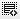How to calculate the stock beta?0
How to solve this question:
The total risk of Stock Y is 0.2, the total risk of the index is .18. The covariance of returns between the stock and index is .27, what is the stock’s beta?0

Stock beta= Covariance/ Variance of index.
=(.27/.18*.18)
Click on thisicon to add code snippet.